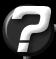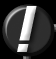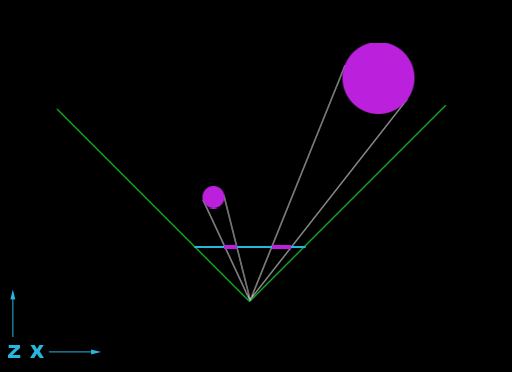Plotting A 3D Point On A 2D Screen Question submitted by (18 June 2001)Return to The ArchivesI have a series of points with the coordinates in 3 arrays x,y and z.The viewpoint of the observer is always constant (imagine a person sat in front of a TV, his head is aligned with the centre of the screen, but just a little way back from it).I need to plot all these 3d points on the screen, but I have no idea what the trigonometrical expression would look like. Would you be able to help me with that? I just need an equation, not any actual language-specific syntax.I'd be very grateful for any help you could give me.Many thanks, Adam WardThe answer to this is a little more in-depth than you might think, and beyond that, it can get quite complex (diving into homogenous 4-Dimensional "perspective space", if you want to get specific.) However, there is a quick and dirty answer to get you up and running in short order. I'll cover a little bit about the background as well, to help you get accustomed to 3D.

Specifically, what you're asking about is called "perspective projection". The concept is to project a 3D image onto a 2D screen, maintaining the look of perspective (i.e. the foreshortening effect you get when things are farther away, they look smaller.)

Let's consider figure 1:Let's start with a few definitions. There's the viewpoint (this is where your 3D camera is located) – in terms of figure 1, it's at the apex where the two green lines meet. Those green lines define your "view frustum" as seen from above. In reality, this frustum is a four-side pyramid. Each side of the pyramid represents the extents that the viewer can see in each of the four directions (left, right, up, down.) The shape of the pyramid suggests that the "extents" I'm referring to get wider and wider as the distance grows (away) from the viewpoint.

The frustum is a pyramid for a very practical reason. Consider standing in a field holding a picture frame out in front of you at arms length. If somebody were to walk away from you only a few meters, they would only be able to step side to side a few times in order to stay inside the picture frame. But if they walk out 100 meters, they would have step sideways a lot farther in order to step out of frame. Of course, you'll also notice that the farther away they walk, the smaller they appear in frame.

Our goal here (as you stated) is to figure out where a point in space would be when projected onto the screen (the picture frame.) This can be thought of as a two-pass operation. Once for X and once for Y (the two points needed in order to plot a point on a 2D screen.) Figure one shows the first pass: calculating X. So figure one is a view from directly above, where the Z coordinate extends away from the viewpoint (negative Z extends behind the viewpoint) and X extends to the right (with negative X extending to the left.) To clarify, a point directly in front of the viewpoint would have an X coordinate of zero, and a Z coordinate that is the distance from the point to the viewpoint. The goal is to project the two groups of points (which are arranged in a circular fashion in figure 1) onto the blue line (a.k.a. the screen, a.k.a. "projection plane").

If this sounds complicated, sit tight. It should start to make sense soon.

I we were to re-state the obvious in shorter terms, we could say that the size of an object (a set of 3D points) gets smaller as the distance to the camera gets larger. In other words, we are performing a simple scale operation based on the distance. Scaling translates to multiplication and division, and the distance is the Z coordinate of the point.

So...

 ``` x_screen = x_3D / z_3D;// The same goes for the Y coordinate: y_screen = y_3D / z_3D; ```

But we're not done just yet. Those two coordinates (x_screen/y_screen) are relative to the center of the screen. Remember, a person walking in the field that hasn't stepped to one side or the other is in the center of the frame. And 2D graphics are relative to the upper-left of the screen, not the center. Also, the typical 2D coordinate system is "X goes to the right and Y goes down." But in 3D, X goes to the right and Y goes up. So we need to negate the Y coordinate, or everything will be upside down. This translates to:

 ``` half_screen_width = screen_width / 2; half_screen_height = screen_height / 2; x_screen = +(x_3D / z_3D) + half_screen_width; y_screen = -(y_3D / z_3D) + half_screen_height; ```

Since we're talking about relativity, it's important to know something else: This calculation only works when your 3D points are relative to your 3D viewpoint. This is always the case if your viewpoint is located at [0,0,0]. But if not, you'll need to do some subtraction. Simply subtract each of the points' coordinates from the viewpoint's coordinates, like so:

 ``` x_3D = input_x – viewpoint_x; y_3D = input_y – viewpoint_y; z_3D = input_z – viewpoint_z; ```

We've got a couple things left: First is perspective scale. Consider a point that has a Z value of 100 (in front of the viewpoint) and an X value of 100 (to the right of the viewpoint.) According to figure 1, which shows a 90-degree frustum – 45-degrees to either side, that point should be someplace on the right-hand side green line. And if it's on the green line, the point should project to one of the points on the far right edge of the screen. But if we divide 100 by 100, we get 1.0. If you add the screen-center to that point, you'll end up with a point very close to the center of the screen. So we need to scale one more time, by half of the width & height of the screen.

 ``` half_screen_width = screen_width / 2; half_screen_height = screen_height / 2; x_screen = (+(x_3D / z_3D) + half_screen_width) * half_screen_width; y_screen = (-(y_3D / z_3D) + half_screen_height) * half_screen_height; ```

While I'm at it, I'm going to cover aspect as well. Aspect is a term that ratio of how wide the screen is, as compared to its height. With a perfectly square monitor (width = height) and a square video resolution (512x512) the aspect ratio will be 1:1. Life is rarely that simple, and graphics never is. Monitors are typically 1.3:1 (i.e. the width of your monitor is 1.3 times wider than the height – measure it and see for yourself. :) Fortunately, the standard video resolutions have a matching aspect. To validate this, calculate any of these: 320/240 or 640/480 or 800/600 or 1024/768 or 1600x1200 or 2048x1536. Due to these aspect ratios being the same (the monitor and the video mode) they cancel each other out.

However, you're most likely going to be rendering your graphics to a window. And if you allow your window to be resized, the user can choose any size they want. For a window to maintain the same convenient aspect ratio as the screen resolution (provided you're using one of the standard resolutions with a 1.3:1 ratio), your window must also have a width:height ratio that is 1.3:1. If not, the solution is quite simple. You need to apply one final scale to your screen coordinates. Here's a typical aspect ratio calculation:

 ``` window_aspect = window_width / window_height; if (window_aspect > 1.0) { x_screen = x_screen / window_aspect; } else { y_screen = y_screen * window_aspect; } ```

If you're simply trying to get some 3D displayed in your application (for graphs, etc.) then this explanation should suffice. However, if you plan on diving into 3D seriously, you should study up on 3D transformations. As I stated earlier, this can get rather complex but in the end it simplifies things tremendously, while giving you a lot more control over the display of your 3D world. This is important, because the transformation pipeline can combine a series of different operations in a single transform. As your tasks get more complex, it will be harder to keep track of what's going on (and difficult to keep efficient.)Response provided by Paul Nettle